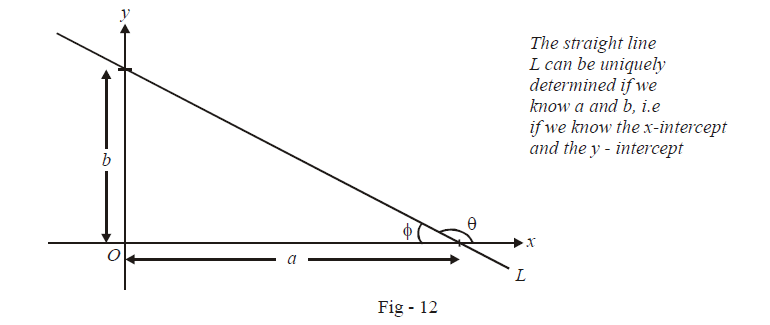# Intercept Form of a Straight Line Equation

Go back to  'Straight Lines'

Lets discuss another form. Notice that to uniquely determine any straight line, we either need the slope of the line and a point through which this line passes, or we need at least two points through which that line passes. Thus for example, a line can also be uniquely determined if we are given the two points where this line intersects the x-axis and the y-axis.Notice that  \begin{align}\tan \phi = \frac{b}{a}\end{align} so that the slope of the line is m = \tan \theta = \,\,\tan \left( {\pi - \phi } \right) = - \tan \phi = \begin{align}- \frac{b}{a}\end{align}. Also, the y-intercept is b. Thus, using the slope intercept form obtained earlier, the equation of the line L is

\begin{align} &\qquad\quad y = - \frac{b}{a}x + b \\& \Rightarrow \quad bx + ay = ab \\& \Rightarrow \quad \boxed{\,\frac{x}{a}\,\, + \,\,\frac{y}{b} = 1\,}\,\,\,\,\,\,\,\,\,\,\,\,\,\,\,\,\,\,\,\,:{\;\mathbf{Intercept}}{\text{ }}{\mathbf{form}} \\& \,\,\,\,\,\,\,\,\,\,\,\,\,\,\, \nearrow \,\,\,\,\,\,\,\,\,\quad \nwarrow \\& x - {\text{intercept}}\quad y - {\text{intercept}} \end{align}

Thus, if we know the x and y intercepts, we can directly use this form to write the equation of the line.